# Content:  Java Program Examples Part 1

Java Program Examples

The following section provides some Java program examples. It is for your exercises, getting to be familiar with basic Java programming and using the jGRASP in a fast track manner. The format is in questions and answers and some solutions provide flowcharts as a simple algorithm. It will try to cover the Java fundamental programming, data types, operators, program controls, array and up to basic object oriented programming. Enjoy.

1.        Write a program that reads an integer and checks whether it is odd or even.  For example:

Enter a number:25

25 is an odd number.

The following is an algorithm for this program using a flow chart. We can use a modulus operator to solve this problem. There will be no remainder for even number when we modulus number by 2.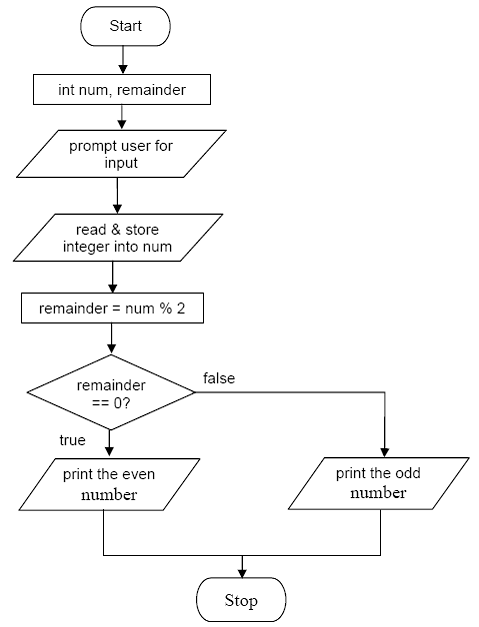The source code:

 import java.util.Scanner; publicclass OddEvenNumber {       public static void main(String[] args)       {          int num, remainder;                  Scanner readinput = new Scanner (System.in);          // read and store input from user         System.out.print ("Enter an integer: ");         num = readinput.nextInt();         // find the remainder         remainder = num % 2;         // test remainder for odd or even        if(remainder == 0)           System.out.println(num + " is an even number.");         else            System.out.println(num + " is an odd number.");   }}

An output samples:

 Enter an integer: 100100 is an even number. Enter an integer: -13-13 is an odd number. Enter an integer: 5151 is an odd number. Enter an integer: 00 is an even number.

2.        The wind chill index (WCI) is calculated from the wind speed`v` in miles per hour and the temperature `t` in Fahrenheit. Three formulas are used, depending on the wind speed:

`if (0 <= v <= 4) then WCI = t`
`if (v >=45) then WCI = 1.6t - 55`
`Otherwise, WCI = 91.4 + (91.4 - t)(0.0203v - 0.304(v)1/2 - 0.474)`

Write a program that can calculate the wind chill index.

The if-else is suitable for this solution, choosing from three conditional expressions. We need to prompt user for v and t in order to calculate the wci. The following is an algorithm for this program using a flow chart.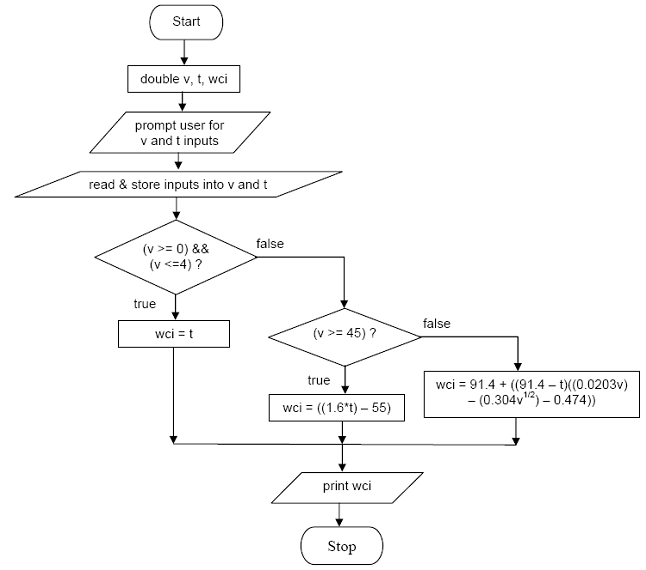The source code:

 import java.util.Scanner;// for pow(x,y)import java.lang.Math;// for double formattingimport java.text.DecimalFormat; publicclass WindChillIndex {       public static void main(String[] args)       {          double v, t, wci;                   // instantiate new object of type Scanner         Scanner readinput = new Scanner (System.in);          // prompt user for wind speed         System.out.print("Enter wind speed in mph: ");         // read and store wind speed         v = readinput.nextFloat();         // prompt user for temperature and store it         System.out.print("Enter temperature in Fahrenheit: ");         t = readinput.nextFloat();         // test wind speed to calculate wci        // for 0 <= v <= 4        if((v >= 0) && (v <=4))            wci = t;         // for v >= 45        else if (v >= 45)             wci = ((1.6*t) - 55);          // others        else            wci = 91.4 + ((91.4 - t)*((0.0203*v) - (0.304*(Math.pow(v,0.5))) - 0.474));                  // do some double formatting         DecimalFormat fmt = new DecimalFormat("0.##");         System.out.println("For wind speed = " + fmt.format(v) + " and "  + "temperature = " + fmt.format(t) +":");         // print wci         System.out.println("Wind Chill Index is: " + fmt.format(wci));       } }

An output samples:

 Enter wind speed in mph: 15Enter temperature in Fahrenheit: 75.2For wind speed = 15 and temperature = 75.2:Wind Chill Index is: 69.58 Enter wind speed in mph: 53Enter temperature in Fahrenheit: 63.5For wind speed = 53 and temperature = 63.5:Wind Chill Index is: 46.6 Enter wind speed in mph: 3.5Enter temperature in Fahrenheit: 88.7For wind speed = 3.5 and temperature = 88.7:Wind Chill Index is: 88.7

3.        Write a program that asks the user to enter an integer and determines whether it is divisible by 5 and 6, whether it is divisible by 5 or 6, and whether it is divisible by 5 or 6 but not both. For example, if your input is 10, the output should be:

Is 10 divisible by 5 and 6? false

Is 10 divisible by 5 or 6? true

Is 10 divisible by 5 or 6, but not both? true

We can use the logical AND (&&), OR (||), NOT (!) and modulus (%) to solve this problem. If the modulus yields a 0, the number is divisible otherwise it is not divisible. Then we use the logical operators to provide the desired outputs. The following is an algorithm for this program using a flow chart.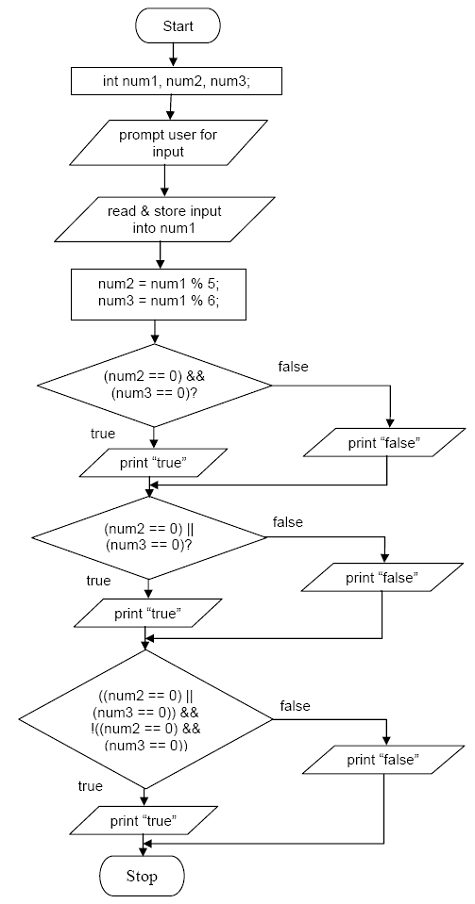The source code:

 import java.util.Scanner; publicclass DivisibleOrNot {       public static void main(String[] args)       {          int num1, num2, num3;                  Scanner readinput = new Scanner(System.in);                   System.out.print("Enter an integer: ");         num1 = readinput.nextInt();                  // Let determine the divisibility of 5 and 6         num2 = num1 % 5;         num3 = num1 % 6;                  System.out.println();                  // Divisible by 5 AND 6?        // using equality and logical operators        if((num2 == 0) && (num3 == 0))         {            System.out.println("Is " + num1 + " divisible by 5 AND 6? true");         }         else         {            System.out.println("Is " + num1 +  " divisible by 5 AND 6? false");         }                  // Divisible by 5 OR 6?        if((num2 == 0) ||(num3 == 0))         {            System.out.println("Is " + num1 + " divisible by 5 OR 6? true");         }         else         {            System.out.println("Is " + num1 +  " divisible by 5 OR 6? false");         }                  // Divisible by 5 OR 6 but NOT both?        if(((num2 == 0) ||(num3 == 0)) && !((num2 == 0) && (num3 == 0)))         {            System.out.println("Is " + num1 + " divisible by 5 OR 6 but NOT both? true");         }         else         {            System.out.println("Is " + num1 +  " divisible by 5 OR 6 but NOT both? false");         }      }}

An output samples:

 Enter an integer: 5 Is 5 divisible by 5 AND 6? falseIs 5 divisible by 5 OR 6? trueIs 5 divisible by 5 OR 6 but NOT both? true Enter an integer: 6 Is 6 divisible by 5 AND 6? falseIs 6 divisible by 5 OR 6? trueIs 6 divisible by 5 OR 6 but NOT both? true Enter an integer: 30 Is 30 divisible by 5 AND 6? trueIs 30 divisible by 5 OR 6? trueIs 30 divisible by 5 OR 6 but NOT both? false  // this is OK or not?Enter an integer: 0 Is 0 divisible by 5 AND 6? trueIs 0 divisible by 5 OR 6? trueIs 0 divisible by 5 OR 6 but NOT both? false Enter an integer: 1 Is 1 divisible by 5 AND 6? falseIs 1 divisible by 5 OR 6? falseIs 1 divisible by 5 OR 6 but NOT both? false

4.        MyJava Café wants you to write a program to take orders from the Internet. Your program asks for the item, its price, and if overnight shipping is wanted. Regular shipping for items under \$10 is \$2.00; for items \$10 or more shipping is \$3.00. For overnight delivery add \$5.00.  For example, the output might be:

`Enter the item:`
`Tuna Salad`
`Enter the price:`
`450`
`Overnight delivery (0==no, 1==yes):`
`1`
`Invoice:`
`  Tuna Salad    4.50`
`  shipping      7.00`
`  total         11.50`

Using the nested if-else, we test the overnight delivery condition that chosen by user. After confirming the overnight delivery, on the true path, we test the amount of price whether it is less than \$10 or not. On the false side, we also test the price whether less than \$10 or not and finally print the total price for the respective condition. The following is an algorithm for this program using a flow chart.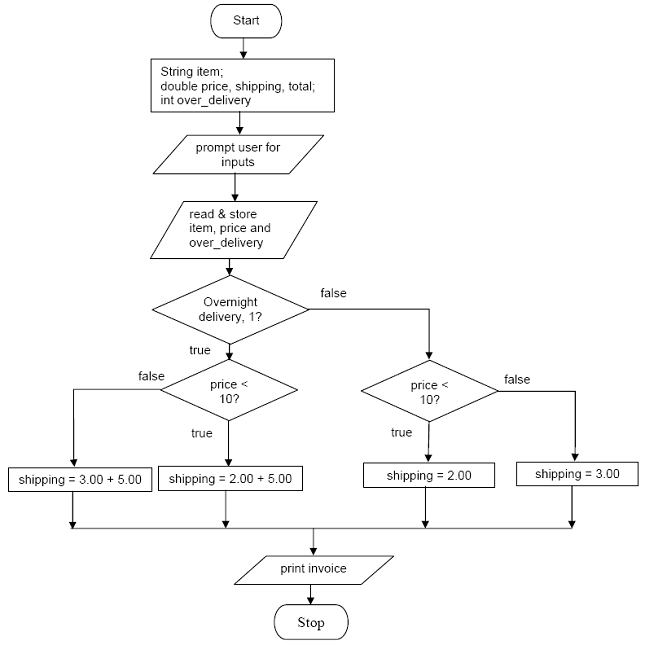The source code:

 import java.util.Scanner; publicclass MyJavaCafeDelivery {       public static void main(String[] args)       {          String item = "";         double price = 0.0, shipping = 0.0, total = 0.0;          int over_delivery;                   // instantiate new object of type Scanner class         Scanner readinput = new Scanner(System.in);          // read and store input into item         System.out.print("Enter the item: ");         item = readinput.nextLine();         // read and store input into price         System.out.print("Enter the price (\$): ");         price = readinput.nextDouble();         // read and store input into over_delivery         System.out.print("Overnight delivery (0==No, 1==Yes): ");         over_delivery = readinput.nextInt();         // test using nested if-else        if(over_delivery == 1)         {            if(price < 10)               shipping = 2.00 + 5.00;            else               shipping = 3.00 + 5.00;         }         if (over_delivery == 0)          {             if(price < 10)               shipping = 2.00;            else               shipping = 3.00;         }         // print all info         System.out.println("Invoice (in \$):");         System.out.println("    "+item+"    "+price);         System.out.println("    shipping    "+shipping);         total = price + shipping;         System.out.println("    total       "+total);      }}

An output samples:

 Enter the item: Tuna SaladEnter the price (\$): 3.99Overnight delivery (0==No, 1==Yes): 1Invoice (in \$):            Tuna Salad              3.99            shipping                7.0            total                   10.99 Enter the item: Potato chipEnter the price (\$): 11.25Overnight delivery (0==No, 1==Yes): 1Invoice (in \$):            Potato chip             11.25            shipping                8.0            total                   19.25 Enter the item: Orange juiceEnter the price (\$): 8.90Overnight delivery (0==No, 1==Yes): 0Invoice (in \$):            Orange juice            8.9            shipping                2.0            total                   10.9 Enter the item: Lemon cakeEnter the price (\$): 12.50Overnight delivery (0==No, 1==Yes): 0Invoice (in \$):            Lemon cake              12.5            shipping                3.0            total                   15.5

5.        Write a program that reads an integer between 0 – 999 and adds all the digits in the integer. For example, if an integer is 932, the sum of all its digit is 14. Hint: Use the % operator to extract digits and use the / operator to remove the extracted digit. For instance, 932 % 10 = 2 and 932 / 10  = 93.

The sum of integer digits is the sum of the remainder when the integer is repeatedly modulus’ed by 10 and then divided by 10 until the integer becomes 0. The following is an algorithm for this program using a flow chart.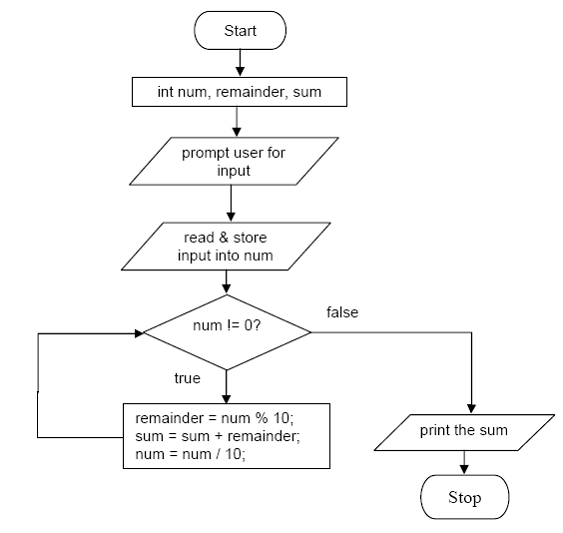import java.util.Scanner; publicclass SumOfDigit {       public static void main(String[] args)       {          int num = 0, remainder = 0, sum = 0;          // instantiate new object of type Scanner class         Scanner readinput = new Scanner(System.in);          // read and store an integer into num         System.out.print("Enter integer between 0 and 999: ");         num = readinput.nextInt();         // print sum of digit         System.out.print("The sum of digits in "+num+" is ");         // using while loop        while(num != 0)         {            // get the remainder by dividing by 10           // the remainder is the digit            remainder = num % 10;            // sum up the remainder            sum = sum + remainder;            // divide the number by 10 to move to the           // next...1000, 100, 10, 1            num = num / 10;         }         System.out.print(sum);      }}

An output samples:

 Enter integer between 0 and 999: 998The sum of digits in 998 is 26 Enter integer between 0 and 999: 100The sum of digits in 100 is 1 Enter integer between 0 and 999: 111The sum of digits in 111 is 3 Enter integer between 0 and 999: 505The sum of digits in 505 is 10